Next: Classification Up: Regression Previous: Gaussian mixture regression (cluster   Contents

### Support vector machines and regression

Expanding the regression function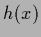in a basis of eigenfunctions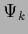of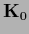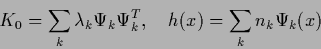(333)

yields for functional (247)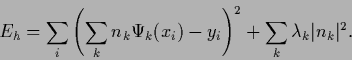(334)

Under the assumption of output noise for training data the data terms may for example be replaced by the logarithm of a mixture of Gaussians. Such mixture functions with varying mean can develop flat regions where the error is insensitive (robust) to changes of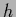. Analogously, Gaussians with varying mean can be added to obtain errors which are flat compared to Gaussians for large absolute errors. Similarly to such Gaussian mixtures the mean-square error data term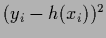may be replaced by an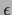-insensitive error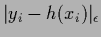, which is zero for absolute errors smallerand linear for larger absolute errors (see Fig.5). This results in a quadratic programming problem and is equivalent to Vapnik's support vector machine [225,74,226,214,215,49]. For a more detailed discussion of the relation between support vector machines and Gaussian processes see [229,208].Next: Classification Up: Regression Previous: Gaussian mixture regression (cluster   Contents
Joerg_Lemm 2001-01-21